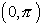Inverse Trig Functions
(also see Composition of Inverse Trig Functions)

Inverse trig functions are also referred to as arcfunctions.  The notation may be: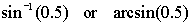where each is interpreted as "the angle whose sine is 0.5".

 Find sin-1(0.5) in degrees

Set the MODE to Degree.      Type on the Home Screen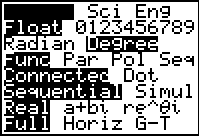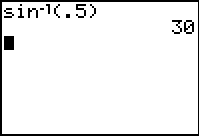The angle whose sine is 0.5 is 30ş.  But it could also be 150ş, 390ş, 510ş, ...
If the problem was stated as Arcsin(0.5), where the A was capitalized,
it would have been asking for the function Arcsin in the interval [-90ş, 90ş]
instead of the multi-valued relation arcsin.
When the notation is sin-1(0.5), you will need to read the context of the problem.

 Find sec-1(3/2) in degrees

Be careful when working with the inverses of the reciprocal trig functions.  Their computations may not be what you expect them to be.  Observe:

Arc notation                                                   Exponent notation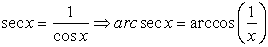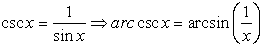---------------------------------------------------------- Be careful with cotangent inverse! If we try to use this same technique with cotangent inverse, stating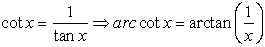, we get a formula that does not yield all possible results.  For example, unlike the inverses of secant and cosecant, cotangent inverse deals with angles whose cotangent may be zero.  It is preferable to state a more universal formula that works in all cases, such as :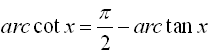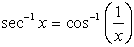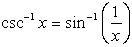---------------------------------------- Another complete formula for cotangent inverse can be: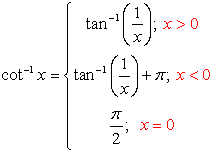OR use the previous formula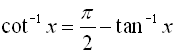S O L U T I O N:
Find sec-1(3/2) in degrees.

Set the MODE to Degree.      Type on the Home Screen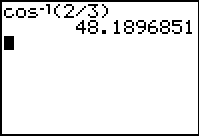Graph the inverse trig functions

The graphing calculator is programmed to graph arcsin(x), arccos(x) and arctan(x) as functions (thus the domains are limited to ensure that the graphs pass the vertical line test for functions).f (x) = sin-1(x) f (x) = arcsin(x)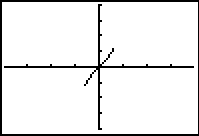Domain: [-1,1] Range: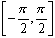f (x) = cos-1(x) f (x) = arccos(x)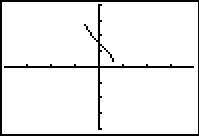Domain: [-1,1] Range: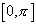f (x) = tan-1(x) f (x) = arctan(x)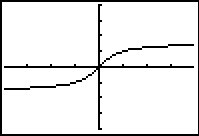Domain: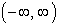Range: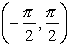f (x) = csc-1(x) f (x) = arccsc(x)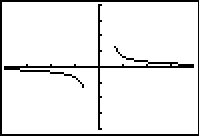Domain: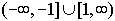Range: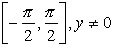f (x) = sec-1(x) f (x) = arcsec(x)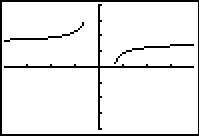Domain:Range: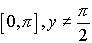f (x) = cot-1(x) f (x) = arccot(x)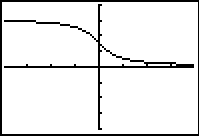Domain:Range: